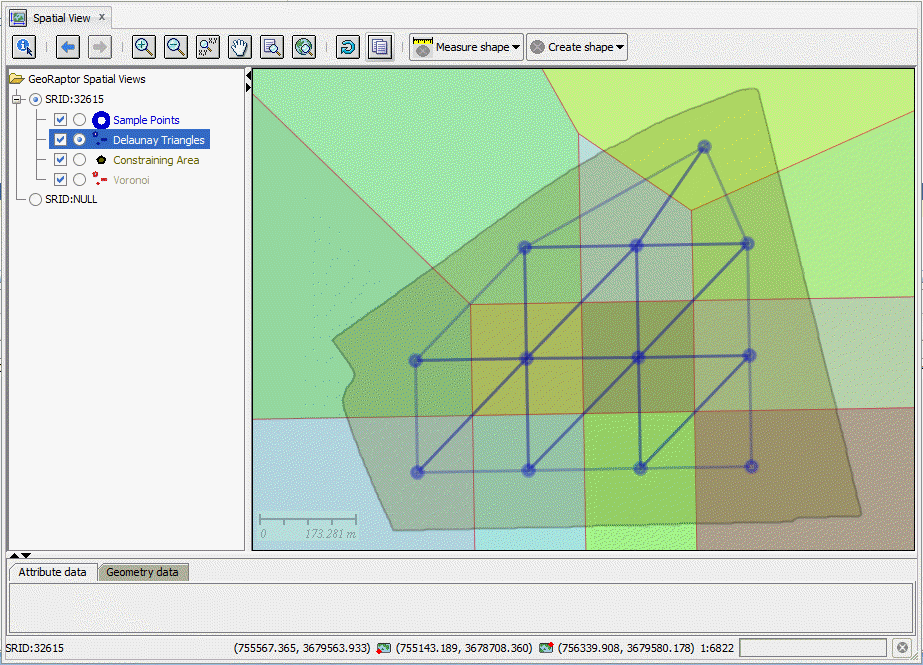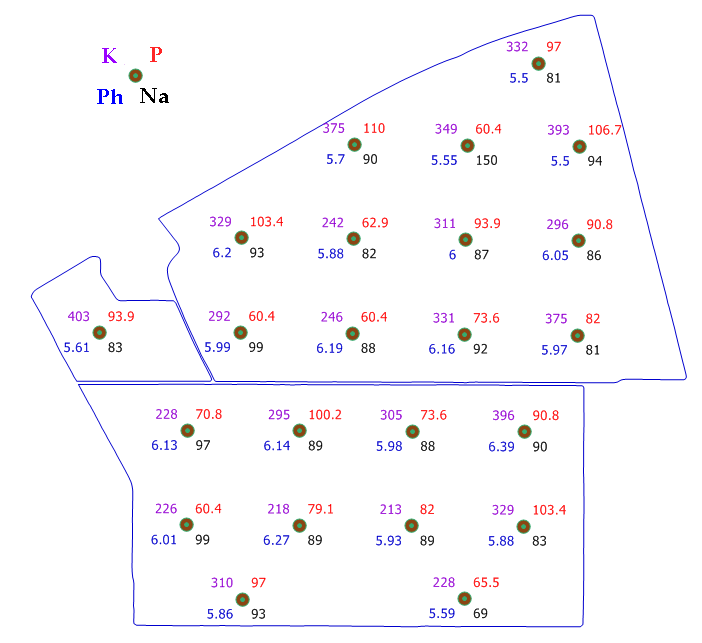# Application of Delaunay Triangulation and Inverse Distance Weighting (IDW) in Oracle for Soils Interpolation

## Introduction

The need to be able to create and use Delaunay triangles (or voronoi diagrams) inside a database is something not often heard. In addition, neither is Z interpolation of grid cell values using Delaunay triangles in consort with an Inverse Distance Weighted (IDW) algorithm.

Why would you want to?” is a common cry.

And, for many years I would have agreed with you but recently two things intersected in a most unexpected way.

### 1. SC4O API Project

In the SC4O project, I decided to expose JTS’s ability to create Delaunay triangles (and Voronoi cells) from a set of input vertices (I have seen talk of the need for this in the PostGIS discussion list but no solution, integrated into the PostGIS API is yet available). I have also exposed the ability to interpolate Z values from a Delaunay triangle. These exposed functions are:

``` define defaultSchema='&1'

CREATE OR REPLACE package SC4O
AUTHID CURRENT_USER
AS

/**
* ST_DelaunayTriangles
* Method for creating a delaunay triangulation from a geometry input (eg multipoints)
*
* @param p_geometry    : mdsys.sdo_geometry : Single sdo_geometry object from whose vertices the delaunay triangles will be build.
* @param p_tolerance   : number             : Snapping tolerance which will be used to improved the robustness of the triangulation computation.
* @param p_precision   : Number             : number of decimal places of precision when comparing ordinates.
* @return SDO_GEOMETRY : Collection of Polygon geometries.
* @history Simon Greener, February 2012, Original Coding
*/
FUNCTION ST_DelaunayTriangles(p_geometry  IN mdsys.sdo_geometry,
p_tolerance IN NUMBER,
p_precision IN NUMBER)
RETURN mdsys.sdo_geometry
Deterministic;

/**
* ST_DelaunayTriangles
* Method for creating a delaunay triangulation from a geometry input (eg multipoints)
*
* @param p_resultSet   : Ref Cursor : Selection of sdo_geometry objects from whose vertices the Delaunay Triangles will be built
* @param p_tolerance   : number     : Snapping tolerance which will be used to improved the robustness of the triangulation computation.
* @param p_precision   : Number     : number of decimal places of precision when comparing ordinates.
* @return SDO_GEOMETRY : Collection of Polygon geometries.
* @history Simon Greener, February 2012, Original Coding
*/
FUNCTION ST_DelaunayTriangles(p_resultSet IN &&defaultSchema..SC4O.refcur_t,
p_tolerance IN NUMBER,
p_precision IN NUMBER)
RETURN mdsys.sdo_geometry
Deterministic;

/**
* ST_DelaunayTriangles
* Method for creating a delaunay triangulation from a geometry input (eg multipoints)
*
* @param p_geomset     : sdo_geometry_array : Array of sdo_geometry objects from whose vertices the delaunay triangles will be build.
* @param p_tolerance   : number             : Snapping tolerance which will be used to improved the robustness of the triangulation computation.
* @param p_precision   : Number             : number of decimal places of precision when comparing ordinates.
* @return SDO_GEOMETRY : Collection of Polygon geometries.
* @history Simon Greener, February 2012, Original Coding
*/
FUNCTION ST_DelaunayTriangles(p_geomset   IN mdsys.sdo_geometry_array,
p_tolerance IN NUMBER,
p_precision IN NUMBER)
RETURN mdsys.sdo_geometry
Deterministic;

/**
* ST_Voronoi
* Method for creating a Voronoi diagram from a geometry input (eg multipoints)
*
* @param p_geometry    : mdsys.sdo_geometry : Single geometry containing source points from whom the voronoi will be built.
* @param p_tolerance   : number             : Snapping tolerance which will be used to improved the robustness of the computation.
* @param p_precision   : Number             : number of decimal places of precision when comparing ordinates.
* @return SDO_GEOMETRY : Collection of Polygon geometries.
* @history Simon Greener, February 2012, Original Coding
*/
FUNCTION ST_Voronoi(p_geometry  IN mdsys.sdo_geometry,
p_tolerance IN NUMBER,
p_precision IN NUMBER)
RETURN mdsys.sdo_geometry
Deterministic;

/**
* ST_Voronoi
* Method for creating a Voronoi diagram from a selection of geometry objects.
*
* @param p_resultSet   : Ref Cursor : Selection (cursor) of sdo_geometry objects from whose vertices the voronoi will be built.
* @param p_tolerance   : number     : Snapping tolerance which will be used to improved the robustness of the triangulation computation.
* @param p_precision   : Number     : number of decimal places of precision when comparing ordinates.
* @return SDO_GEOMETRY : Collection of Polygon geometries.
* @history Simon Greener, February 2012, Original Coding
*/
FUNCTION ST_Voronoi(p_resultSet IN &&defaultSchema..SC4O.refcur_t,
p_tolerance IN NUMBER,
p_precision IN NUMBER)
RETURN mdsys.sdo_geometry
Deterministic;

/**
* ST_Voronoi
* Method for creating a Voronoi diagram from an array of geometry objects.
*
* @param p_geomset     : sdo_geometry_array : Array of sdo_geometry objects from whose points the voronoi will be built.
* @param p_tolerance   : number             : Snapping tolerance which will be used to improved the robustness of the triangulation computation.
* @param p_precision   : Number             : number of decimal places of precision when comparing ordinates.
* @return SDO_GEOMETRY : Collection of Polygon geometries.
* @history Simon Greener, February 2012, Original Coding
*/
FUNCTION ST_Voronoi(p_geomset   IN mdsys.sdo_geometry_array,
p_tolerance IN NUMBER,
p_precision IN NUMBER)
RETURN mdsys.sdo_geometry
Deterministic;

/**
* ST_InterpolateZ
* @param p_point : mdsys.sdo_geometry : Point for which Z ordinate's value is to be computed
* @param p_geom1 : mdsys.sdo_geometry : First corner geometry 3D point
* @param p_geom2 : mdsys.sdo_geometry : Second corner geometry 3D point
* @param p_geom3 : mdsys.sdo_geometry : Third corner geometry 3D point
* @return Number : Result of Interpolation
* @throws SQLException
* @history Simon Greener, March 2012, Original Coding
*/
FUNCTION ST_InterpolateZ(p_point IN mdsys.sdo_geometry,
p_geom1 IN mdsys.sdo_geometry,
p_geom2 IN mdsys.sdo_geometry,
p_geom3 IN mdsys.sdo_geometry)
RETURN NUMBER Deterministic;

/**
* ST_InterpolateZ
* @param _point         : STRUCT : Point for which Z ordinate's value is to be computed
* @param _facet         : STRUCT : 3 vertex triangular polygon
* @return double        : Result of Interpolation
* @throws SQLException
* @history Simon Greener, March 2012, Original Coding
*/
FUNCTION ST_InterpolateZ(p_point IN mdsys.sdo_geometry,
p_facet IN mdsys.sdo_geometry)
RETURN mdsys.sdo_geometry Deterministic;

END SC4O;
```

An example of some sample points being turned into a set of Delaunay triangles can be seen here:### 2. Customer Question

Someone (now a customer) contacted me asking if I could help build a set of tools inside PostgeSQL/PostGIS for the interpolation/extrapolation of observed values at regular sample points throughout agricultural fields.

The reason the customer contacted me is that he had seen my articles on gridding vector polygons in PostGIS and wondered if this gridding could be extended to include interpolation to code each created grid cell with a value calculated from a set of sample points.

The field, and values observed at each sample point (I only include 4 out of all the variables: Potassium, Phosphorus, Ph and Sodium) are shown in the following diagram.### Bringing both Together

Having thought about it I first looked at the new ST_Raster capabilities of PostGIS 2.0. It has enormous promise (I could create the raster, assign the sample point values the associated cell but…) except for one thing: there is no ability to do any interpolation in the current system through which I could take the limited sample point values and compute and assign values for the other grid cells. It may be my lack of ability with raster processing (likely) but looking at ST_Raster’s ST_MapAlgebraFctNgb didn’t convince me that it would help with the interpolation: I kept coming back to the vector nature of the problem space.

To solve the problem I first needed to (Oracle first, later PostGIS).

• Loaded field border polygons into a single Oracle table;
• Loaded sample points and P, K etc values (as attributes) into a single Oracle table;
• Exposed and loaded the JTS Delaunay Java into the database and created a PL/SQL wrapper over it;
• Exposed and loaded JTS’s Triangle.interpolateZ(geometry) into the database and created a PL/SQL wrapper over it (geometry can be a single point or a multipoint object);
• Modified my TESSELATE.RegularGrid() PL/SQL function to return simple points instead of/or along with an optimised rectangle that describes a single grid.

Then, to solve the problem (via SQL) I did the following:

• Created Delaunay triangles from the sample points with the Z ordinate being the measured value (eg K);
• Created grid cells that cover the field border polygons;
• Used JTS’s InterpolateZ to calculate and assign a grid cell value (eg K) to each grid cell that falls within or on a Delaunay triangle;
• Used an Inverse Distance Weighted (IDW) algorithm (pure SQL sum/count group by) to assign a variable value to each grid cell that falls outside the Delaunay triangles but using the nearest grid cell values assigned in the previous step;
• Finally, “smoothed” the grid by applying a 3×3 window over the now populated grid cells.

All the above was done using vector processing.

The following 4 images show the result of interpolating and assigning the Potassium (K), Phosphorus (P), Ph and Sodium (Na) observed values across the whole sampled areas.

The following query gives one an idea of the number of (10×10 meter) cells created.

``` SELECT CASE WHEN GROUPING(triangle) = 1
THEN 'Total'
WHEN triangle = 'Y'
THEN 'Inside Delaunay - InterpolateZ'
ELSE 'Outside Delaunay - IDW'
END AS CellReport,
COUNT(*),
SUM(sdo_geom.sdo_Area(cell,0.005)) AS sq_m,
MIN(smoothed_K) AS iMin,
MAX(smoothed_K) AS iMax
FROM GIS.field_iterp_k
GROUP BY rollup(triangle);

-- Results
CELLREPORT                     COUNT(*) SQ_M    IMIN  IMAX
------------------------------ -------- ------- ----- -----
Outside Delaunay - IDW         3579     357900  227.8 399.1
Inside Delaunay - InterpolateZ 6637     663700  214.6 397.8
Total                          10216    1021600 214.6 399.1
```

This sort of processing can be executed at any one of three levels in a solutions architecture: data, application, client.

The customer is currently doing this processing using a collection of open source software from a variety of projects glued together via a scripting language.

When the ability to do this processing is available at the data tier (ie inside the database) then what we see is something that tightly links the creation of source data to associated computed data. Databases offer a variety of mechanisms for tightly integrating the two. For example, the creation of new field (polygon) and sample data can drive, automatically, the creation of the derived data either via database triggers (probably linked to an asynchronous script) or through technologies like materialized views. Thus, if an agricultural scientist noted that they had entered the value for a sample variable incorrectly (eg K) then any update to that value in the database could automatically fire the derived data generation. No one needs to remember to re-run a client script or middle tier application program.